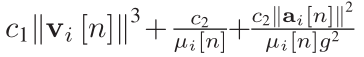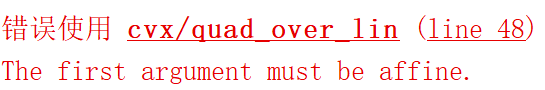Hello,everyone! How do I resolve this formula? we can know variables of the formula are v(2,N) u(1,N) and a(2,N).
my code isI’ll leave out the extra subscripts which you need to handle and fix up.

The norm squared term = a’*a, therefore the last term equals

`c/g^2*quad_over_lin(a,mu)`

quad_over_lin Sum of squares over linear.
Z=quad_over_lin(X,Y), where X is a vector and Y is a scalar, is equal to
SUM(ABS(X).^2)./Y if Y is positive, and +Inf otherwise. Y must be real.

``````If X is a matrix, quad_over_lin(X,Y) is a row vector containing the values
of quad_over_lin applied to each column. If X is an N-D array, the operation
is applied to the first non-singleton dimension of X.

quad_over_lin(X,Y,DIM) takes the sum along the dimension DIM of X.
A special value of DIM == 0 is accepted here, which is automatically
replaced with DIM == NDIMS(X) + 1. This has the effect of eliminating
the sum; thus quad_over_lin( X, Y, NDIMS(X) + 1 ) = ABS( X ).^2 ./ Y.

In all cases, Y must be compatible in the same sense as ./ with the squared
sum; that is, Y must be a scalar or the same size as SUM(ABS(X).^2,DIM).

Disciplined convex programming information:
quad_over_lin is convex, nonmontonic in X, and nonincreasing in Y.
Thus when used with CVX expressions, X must be convex (or affine)
and Y must be concave (or affine).``````

According your problrm, I think the true code can be as following: